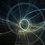# Will someone give me a LOGIC for this??

All of you may have observe that: 3^2+4^2=5^2 (if not then observe it now)

But instead, if you want to find two numbers such that sum of their squares is equals to square of any specific number then it would be a hard task to find them. But I have found myself a general way to find those numbers.

Let T be the number (say 13) for which you want two numbers m,n such that sum of their squares is equals to T^2 (in our example, 13^2=169). Now divide T by 5 and let the answer be a (13/5=2.6). Now:

m=T-a (m=13-2.6=10.4)

n=T-2a (n=13-2x2.6=7.8)

Here we got our numbers. Now check that (m^2+n^2) will be equals to T^2.

Although it worked for every number I have tried yet but I can't find an explanation for that why one have to divide every number with 5. So please comment any logic you can get for this and also your views about should I get an medal or any award for this discovery.Note by Vaibhav Jain
6 years, 7 months ago

This discussion board is a place to discuss our Daily Challenges and the math and science related to those challenges. Explanations are more than just a solution — they should explain the steps and thinking strategies that you used to obtain the solution. Comments should further the discussion of math and science.

When posting on Brilliant:

• Use the emojis to react to an explanation, whether you're congratulating a job well done , or just really confused .
• Ask specific questions about the challenge or the steps in somebody's explanation. Well-posed questions can add a lot to the discussion, but posting "I don't understand!" doesn't help anyone.
• Try to contribute something new to the discussion, whether it is an extension, generalization or other idea related to the challenge.

MarkdownAppears as
*italics* or _italics_ italics
**bold** or __bold__ bold
- bulleted- list
• bulleted
• list
1. numbered2. list
1. numbered
2. list
Note: you must add a full line of space before and after lists for them to show up correctly
paragraph 1paragraph 2

paragraph 1

paragraph 2

[example link](https://brilliant.org)example link
> This is a quote
This is a quote
    # I indented these lines
# 4 spaces, and now they show
# up as a code block.

print "hello world"
# I indented these lines
# 4 spaces, and now they show
# up as a code block.

print "hello world"
MathAppears as
Remember to wrap math in $$ ... $$ or $ ... $ to ensure proper formatting.
2 \times 3 $2 \times 3$
2^{34} $2^{34}$
a_{i-1} $a_{i-1}$
\frac{2}{3} $\frac{2}{3}$
\sqrt{2} $\sqrt{2}$
\sum_{i=1}^3 $\sum_{i=1}^3$
\sin \theta $\sin \theta$
\boxed{123} $\boxed{123}$

Sort by:

However your way is not the only way. Im surprised you found such a specific method. The method I use is basically like an isosceles right triangle. If there is a right triangle that has two legs equal to each other then the hypotenuse is the leg length multiplied by root 2. For example if the there is a right triangle with both legs of side 3 then the hypotenuse is 3(2^0.5) which is 3 times square root of 2. So, if you have only the hypotenuse, you can get two legs easily by dividing the hypotenuse by root 2. So, if in your scenario if the hypotenuse is 13, then two sides can be 13 divided by square root of 2.

- 6 years, 7 months ago

You're basically exploiting the fact that $(3k)^2 + (4k)^2 = (5k)^2$. (This is your identity multiplied by $k^2$.)

From your formula, $m = T - a = T - \dfrac{T}{5} = \dfrac{4T}{5}$ and $n = T - 2a = T - \dfrac{2T}{5} = \dfrac{3T}{5}$

and therefore $m^2 + n^2 = \dfrac{4T}{5}^2 + \dfrac{3T}{5}^2 = \dfrac{5T}{5}^2 = T^2$.

You can do this using other Pythagorean triplets as well, for example, for some number $S$, let $b = \dfrac{S}{13}$. and defining $k = S - b$ and $l = S - 8b$, we can see that $k^2 + l^2 = S^2$

- 6 years, 7 months ago